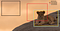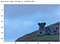# Day 81 (DL) — All about IOU (Interpretation, Visualization, and Evaluation)Photo by Isaac Smith on Unsplash

Intersection Over Union(IOU) is one of the evaluation criteria implemented for object detection use cases. One of the outputs of the object detection algorithm is bounding box coordinates(regressor output), which have to be compared with the ground truth. Totally we have four values as an output corresponding to a single BB box. So how do we compare it with the expected output coordinates?. If the output is just one continuous number(regression scenarios), then we can employ mean absolute error or mean squared error for the comparison.

Since we have 4 coordinate values, we compare the overlap between the boxes with the union of two boxes (i.e) intersection/union(IOU). If the union and intersection values are the same, then the IOU = 1 which is the highly desirable value. Let’s gain intuition with an image.Photo by Ramon Vloon on Unsplash

In the above picture, box1 has no overlap with the ground truth resulting in IOU = 0. Box2 has 40% overlap while box3 has 99% overlap giving IOU as 0.99(preferred outcome). So, one of the principal objectives of the detection algorithm is to boost up the IOU value. Let’s go into the technical interpretation of the bounding box values and how we can visualize it using OpenCV.

Interpretation & Visualization: The width of the image is 4291 and the height is 3285 (4291 x 3285) . The bb box coordinates of the interested object is xmin = 2806.7, ymin = 1573.3, xmax = 4738.73 and ymax = 2831.92. We can use opencv to read the image and display the bounding box in the image.

`import cv2import matplotlib.pyplot as pltimage = cv2.imread('ramon-vloon-OYq3l_mbTxY-unsplash.jpg')image.shape(3285, 4921, 3)`

After reading the image, we can superimpose the bounding box coordinates on it.

`x_min = int(2806.7)y_min = int(1573.3)x_max = int(4738.73)y_max = int(2831.92)image1 = cv2.rectangle(img=image, rec = (x_min, y_min, x_max - x_min, y_max - y_min), color = (0, 255, 0), thickness=10)plt.figure(figsize = (10,10))plt.imshow(image1, 'gray')`Fig 1 — shows image with the bounding box.

As mentioned above let’s consider three cases and compute the IOU for the predicted boxes against the actual bb box. The bounding box values are uploaded into excel.

`bb_box = pd.read_excel('bounding box.xlsx')bb_box`

Let’s display all of the coordinate values,

`for i, row in bb_box.iterrows():    xmin = int(row['xmin'])    ymin = int(row['ymin'])    xmax = int(row['xmax'])    ymax = int(row['ymax'])            image1 = cv2.rectangle(img=image, rec = (xmin, ymin, xmax - xmin, ymax - ymin), color = (0, 255, 0), thickness=10)        plt.figure(figsize = (10,10))plt.imshow(image1, 'gray')`

Evaluation: We can compute the IOU using the ground truth and the predicted value.

ground truth = (xgmin, ygmin, xgmax, ygmax)
predicted = (xpmin, ypmin, xpmax, ypmax)

diff1 = minimum(xgmax, xpmax) — maximum(xgmin, xpmin)
diff2 = minimum(ygmax, ypmax) — maximum(ygmin, ypmin)

Intersection = diff1 * diff2 (area of the overlap)

gheight = xgmax — xgmin
gwidth = ygmax — ygmin

pheight = xpmax — xpmin
pwidth = ypmax — ypmin

union(total area — intersection) = (gheight * gwidth) + (pheight * pwidth) — Intersection

IOU = Intersection/union

`#let's display all of the coordinate valuesfor i, row in bb_box.tail(3).iterrows():    xpmin = int(row['xmin'])    ypmin = int(row['ymin'])    xpmax = int(row['xmax'])    ypmax = int(row['ymax'])            diff1 = np.minimum(xgmax, xpmax) - np.maximum(xgmin, xpmin)    diff2 = np.minimum(ygmax, ypmax) - np.maximum(ygmin, ypmin)        if diff1 <=0 or diff2 <= 0:        print('The coordinate values:', xpmin, ypmin, xpmax, ypmax)        print('There is no overlap')            else:            intersection = diff1 * diff2                gheight = xgmax - xgmin        gwidth = ygmax - ygmin            pheight = xpmax - xpmin        pwidth = ypmax - ypmin            union = (gheight * gwidth) + (pheight * pwidth) - intersection            IOU = intersection / union         print('\nThe coordinate values:', xpmin, ypmin, xpmax, ypmax)        print('The value of Intersection Over Union:',IOU)        print('\nCoordinate values of the ground truth:', xgmin, ygmin, xgmax, ygmax)`

When we print the results, we could notice the box which is closer to the ground truth yields higher IOU.

`The coordinate values: 100 107 1491 1063There is no overlapThe coordinate values: 2020 893 3681 2322The value of Intersection Over Union: 0.15797307557880275The coordinate values: 2756 1522 4789 2882The value of Intersection Over Union: 0.8790457452041318Coordinate values of the ground truth: 2806 1573 4738 2831`

The entire code can be found in the GitHub repository.

One another critical point to note here is, whenever we resize the image, the corresponding bounding boxes should also get adjusted to the new shape. This is usually done by normalizing the bounding box coordinates(divide by height and width of the respective coordinates) and then multiplying it by the new size.

In the object detection setting, the model outputs ’n’ number of overlapping bounding boxes for the same object. For such scenarios, only the box with higher IOU(against the ground truth) must be retained and the rest of the values should be suppressed.

Recommended video:

https://www.youtube.com/watch?v=ANIzQ5G-XPE

## CodeX

Everything connected with Tech & Code

Everything connected with Tech & Code

Medium is an open platform where 170 million readers come to find insightful and dynamic thinking. Here, expert and undiscovered voices alike dive into the heart of any topic and bring new ideas to the surface. Learn more

Follow the writers, publications, and topics that matter to you, and you’ll see them on your homepage and in your inbox. Explore

If you have a story to tell, knowledge to share, or a perspective to offer — welcome home. It’s easy and free to post your thinking on any topic. Write on Medium